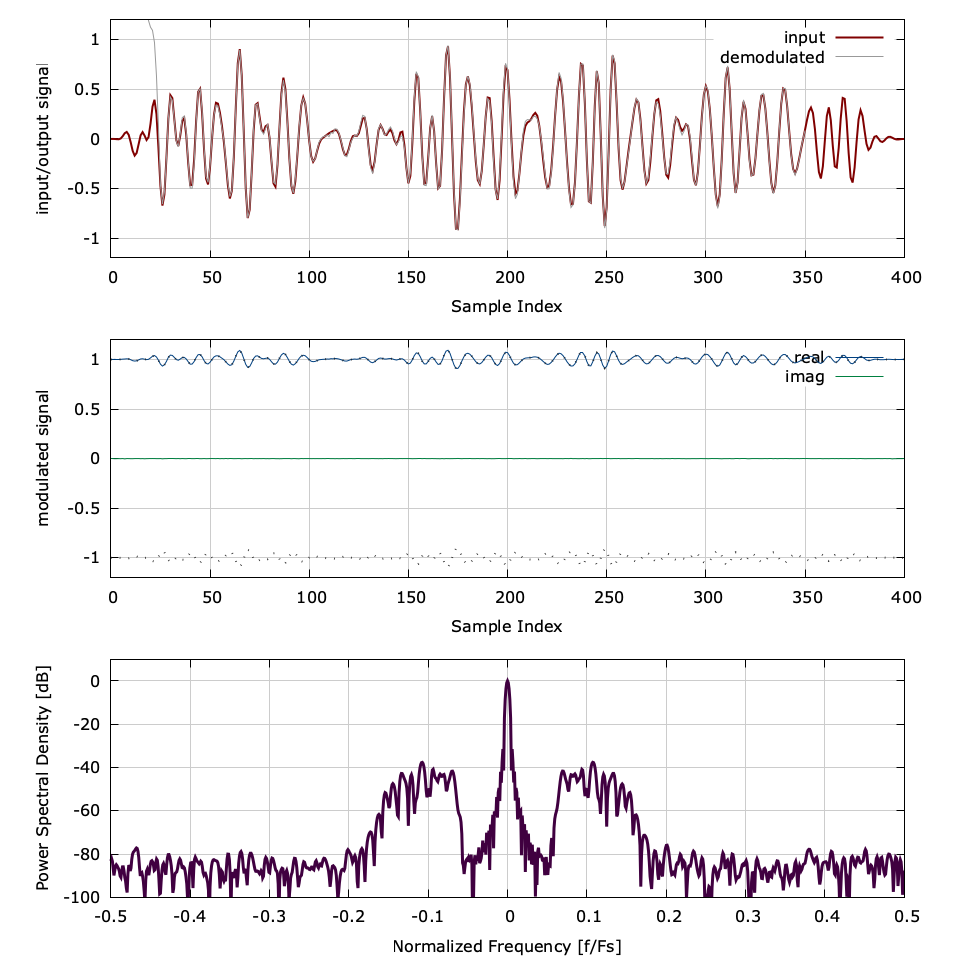# Analog Amplitude Modulator/Demodulator (ampmodem)

The ampmodem object implements an analog amplitude modulation (AM) modulator/demodulator pair. Two basic transmission schemes are available: single side-band (SSB), and double side-band (DSB). For an input message signal $$-1 \le s(t) \le 1$$ , the double side-band transmitted signal is

$$x_{DSB}(t) = \begin{cases} s(t)e^{j 2 \pi f_c t} & \text{suppressed carrier} \\ \frac{1}{2}\bigl(1+ks(t)\bigr)e^{j 2 \pi f_c t} & \text{unsuppressed carrier} \end{cases}$$

where $$f_c$$ is the carrier frequency, and $$k$$ is the modulation index. For single side-band, only the upper (USB) or lower half (LSB) of the spectrum is transmitted. The opposing half of the spectrum is rejected using a Hilbert transform (see [section-filter-firhilb] ). Let $$\dot{s}(t)$$ represent the Hilbert transform of the message signal $$s(t)$$ such that its Fourier transform is non-zero only for positive frequency components, viz

$$\dot{S}(\omega) = \F\left\{ \dot{s}(t) \right\} = \begin{cases} S(\omega) = \F\left\{ s(t) \right\} & \omega > 0 \\ 0 & \omega \leq 0 \end{cases}$$

Consequently the transmitted upper side-band signal is

$$x_{USB}(t) = \begin{cases} \dot{s}(t)e^{j 2 \pi f_c t} & \text{suppressed carrier} \\ \frac{1}{2}\bigl(1+k\dot{s}(t)\bigr)e^{j 2 \pi f_c t} & \text{unsuppressed carrier} \end{cases}$$

For lower single side-band, $$\dot{s}(t)$$ is simply conjugated. For suppressed carrier modulation the receiver uses a phase-locked loop for carrier frequency and phase tracking. When the carrier is not suppressed the receiver demodulates using a simple peak detector and IIR bias removal filter. An example of the freqmodem interface is listed below.


#include <liquid/liquid.h>

int main() {
// options
float mod_index  = 0.1f;                // modulation index (bandwidth)
int   type       = LIQUID_AMPMODEM_USB; // AM type
int   suppressed = 0;                   // suppressed carrier?

// create mod/demod objects
ampmodem mod   = ampmodem_create(mod_index, type, suppressed);
ampmodem demod = ampmodem_create(mod_index, type, suppressed);

float         x;    // original
float complex y;    // modulated
float         z;    // demodulated

// repeat as necessary
{
// modulate sample
ampmodem_modulate(mod, x, &y);

// demodulate sample
ampmodem_demodulate(demod, y, &z);
}

// destroy objects
ampmodem_destroy(mod);
ampmodem_destroy(demod);

return 0;
}


A more detailed example can be found in examples/ampmodem_example.c located under the main liquid project source directory. Listed below is the full interface to the ampmodem object for analog frequency modulation/demodulation.

• ampmodem_create(k,fc,type,suppressed_carrier) creates and returns an ampmodem object with a modulation index $$k$$ , a carrier $$f_c$$ , a modulation scheme defined by type , and a binary flag specifying whether the carrier should be suppressed. The modulation type can either be LIQUID_AMPMODEM_DSB (double side-band), LIQUID_AMPMODEM_USB (single upper side-band), or LIQUID_AMPMODEM_LSB (single lower side-band). method.
• ampmodem_destroy(q) destroys an ampmodem object, freeing all internally-allocated memory.
• ampmodem_reset(q) resets the state of the ampmodem object.
• ampmodem_print(q) prints the internal state of the ampmodem object.
• ampmodem_modulate(q,x,*y) modulates the input sample $$x$$ storing the output to $$y$$ .
• ampmodem_demodulate(q,y,*x) demodulates the input sample $$y$$ storing the output to $$x$$ .Figure [fig-modem-ampmodem]. ampmodem demonstration modulating an audio signal using double side-band, non-suppressed carrier AM with a modulation index of $$k=0.1$$ and a relative carrier frequency $$f_c/F_s = 0.1$$ .

An example of the ampmodem can be found in [fig-modem-ampmodem]


#include <liquid/liquid.h>

int main() {
// options
float mod_index  = 0.1f;                // modulation index (bandwidth)
int   type       = LIQUID_AMPMODEM_USB; // AM type
int   suppressed = 0;                   // suppressed carrier?

// create mod/demod objects
ampmodem mod   = ampmodem_create(mod_index, type, suppressed);
ampmodem demod = ampmodem_create(mod_index, type, suppressed);

float         x;    // original
float complex y;    // modulated
float         z;    // demodulated

// repeat as necessary
{
// modulate sample
ampmodem_modulate(mod, x, &y);

// demodulate sample
ampmodem_demodulate(demod, y, &z);
}

// destroy objects
ampmodem_destroy(mod);
ampmodem_destroy(demod);

return 0;
}